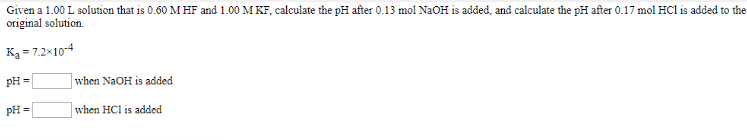# Given a 1.00 L solution that is 0.60 M HF and 1.00 M KF, calculate the pH after 0.13 mol NaOH is added, and calculate the pH after 0.17 mol HCl is added to the original solution. Ka = 7.2 x 10^-4 pH = ___ when NaOH is added pH = ___ when HCl is added